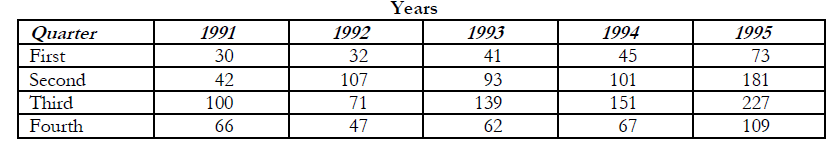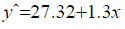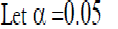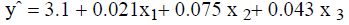# Briefly but clearly, explain the difference, if any, between regression analysis and correlation analysis.

Briefly but clearly, explain the difference, if any, between regression analysis and correlation analysis.Regression analysis checks the study of relationship between variables (independent and dependent).
Correlation analysis on the other hand looks at the strength of relationship of variables. Both regression
and correlation analysis are related since they both look at variables behaviour to each other.
raphael answered the question on January 10, 2019 at 12:14

### Related Questions

• Kenya Graduate School (KGS) offers a variety of graduate courses. However, its main emphasis has been on information science (IS) courses. Due to the laboratory equipment...(Solved)

Kenya Graduate School (KGS) offers a variety of graduate courses. However, its main emphasis has been on
information science (IS) courses. Due to the laboratory equipment requirements for IS courses, KGS has to
estimate in advance the expected students enrolments. Over the last 5 years, the students enrolments, by
quarter, has been:Required:
a) Determine the estimates, by quarter, for year 1996. Justify the method you use.
b) If linear multiple regression were to be used in order to determine the predicting equation, what other
variables would be included?
c) How would the expected enrolments be compared to the actual enrolments?Date posted: January 10, 2019.  Answers (1)

• In recent years environmentalists and health professionals have been concerned about the ill effects onthe environment of the widespread use of insecticides. If human beings...(Solved)

In recent years environmentalists and health professionals have been concerned about the ill effects on
the environment of the widespread use of insecticides. If human beings are to cope with the problem
and make decisions about how to deal with it, they must understand the effect of insecticides on humans
and other animals. In the kind of study often done to promote such understanding, two professors at
University of Nairobi investigated the effect of a commonly used insecticide on sheep. Among other
statistical analyses, they derived the following linear regression equation (n = 16):This equation describes the relationship between the activity of a certain enzyme in the
sheep's brain (Y)and the time (in hours) after the sheep has been exposed to the insecticide (X).
Required:
i) Suppose 30 hours have elapsed since a sheep has been exposed to the insecticide, what is the
predicted value of Y?
ii) How would you describe the relationship between the two variables?iii) The professors computed a coefficient of determination (r2) of 0.86 from the data. What
conclusion can be drawn about the true relationship between the two variables?
iv) What assumption are necessary to solve part (iii) above?

Date posted: January 1, 2019.  Answers (1)

• Does a high correlation mean that one variable causes another variable to vary?(Solved)

Does a high correlation mean that one variable causes another variable to vary?

Date posted: January 1, 2019.  Answers (1)

• Does finding a no linear relationship between two variables mean no relationship?(Solved)

Does finding a no linear relationship between two variables mean no relationship?

Date posted: January 1, 2019.  Answers (1)

• The following regression equation was calculated for class of 24 CPA II students. Required: a) Calculate the t ratio and the 95% confidence interval for each regression...(Solved)

The following regression equation was calculated for class of 24 CPA II students.Standard error (0.0190) (0.034) (0.018)
Where y=students score on a theory examination
x1 = Students rank (from the bottom) in high
school x2 = Students verbal aptitude score
x3 =A measure of students character
Required:
a) Calculate the t ratio and the 95% confidence interval for each regression coefficient.
b) What assumptions did you make in (a) above? How reasonable are they?
c) Which regressor gives the strongest evidence of being statistically discernible?
d) In writing up a final regression equation, should one keep the first regressor in the equation, or drop if?
Why?

Date posted: January 1, 2019.  Answers (1)